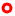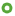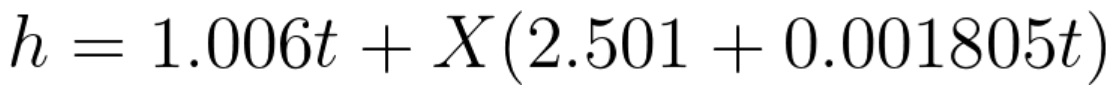# PSYCHROMETRIC CALCULATOR

This psychrometric calculator provides web bulb temperature and other psychrometric calculation in addition to a psychrometric chart. Either wet bulb temperature or relative humidity can be selected as input. You can enter also altitude, that is necessary to make corrections for the atmospheric pressure. A pressure of 1013.25 hPa is considered for sea level (0 m).
After having selected the first point a second point can be generated using the arrows on the diagram below. The table on the left will show main results for both points, and its difference for each parameter.

Temperature and altitude::
 Temperature: ºC Altitude: m

Enter known parameter:

Value:

## Interactive Psychrometric Diagram.Δ
Slow
? Use the arrows to move the second point (green).
Activate 'Slow' option to reduce pointer speed.
Results for both points and its difference are shown on the table at the left.
To change the position of the red point, modify the values above, at the beginning of this page.
Click on "Center" to move the (red) point to the same position than the green. From the new position, you will be able to generate a new green point with the arrows.

RESULTS () :

Presiones
 Barometric Pressure: hPa Vapor pressure: hPa Saturation Vapor Pressure: hPa

Temperatures
 Dry Bulb Temperature: ºC Wet Bulb Temperature: ºC Dew Point: ºC

Humidity
 Relative Humidity: % g of water/kg dry air: g/kg Specific Humidity: Absolute Humidity: g/m3

Others
 Density: kg/m3 Specific Enthalpy: kJ/kg

DEFINITIONS:

The vapor pressure of water is the pressure at which water vapor is in thermodynamic equilibrium with its condensed state.

The pressure of a vapour wich is in equilibrium with its liquid.

It is the temperature measured using a normal thermometer freely exposed to the air but shielded from radiation and moisture.

The wet bulb temperature is the minimum temperature that can be reached by evaporative cooling.
If we try to cool down a solid object with an air flow of, let´s say, 20ºC, the minimum temperature that theoretically we can reach for this solid object is 20ºC. However, if with the same air flow we try to cool down a drop of water, we could reach a lower temperature than 20ºC. The reason is that on the first case there is only one heat transfer mechanism, conduction from the hotter body to the cooler body. In the case of the drop of water, in addition to conduction, some of the water will be evaporated and will pass to the air flow. This process absorbs energy and produce a further reduction of the water temperature. If the air flow rate is big enough we will reach to the wet bulb temperature. Wet bulb temperature depends on how dry is the air. If the relative humidity of the air is 100% (in other words it is saturated with moisture), evaporation will not happen and the wet bulb temperature will be the same than the dry bulb temperature (the one that you can measure with any conventional termometer). On the other hand, if the air has a relative humidity below 100%, some water will evaporate. The drier is the air, the lower the wet bulb temperature.
Wet bulb temperature can be measured by using a thermometer with the bulb wrapped in a water soaked cloth over which enough air is passed.

Relative humidity is the ratio between the amount of water vapour that is contained in the air and the maximum amount of water it could be capable to contain.

Ratio between vapor water mass and the total mass of the humid air.

Also known as water vapor density, is the ratio between the mass of water vapor and total volume.

Ratio between total mass and total volume.

The following equation is used to callculate specifiy enthalpy (kJ/kg):X= mass of water vapour in g for each kg of try air.
t= dry bulb temperature.